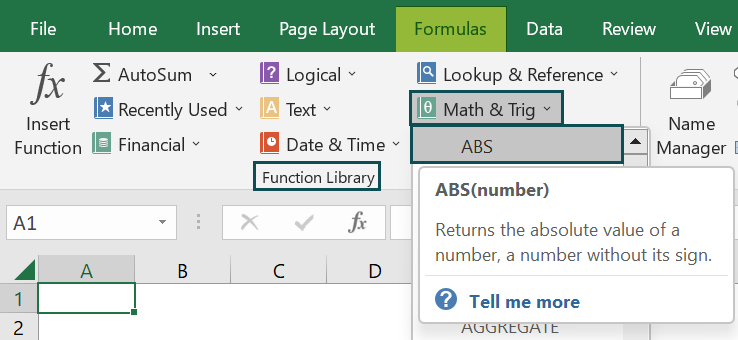# ABS Excel FunctionArticle byExcelMojo Team## What Is ABS Function In Excel?

The ABS function in Excel extracts the numeric value of a selected cell or a cell range and returns the absolute number without the arithmetic sign. If the numeric value is positive, the number itself is returned, and if it is negative, only the number is returned without the minus (-) sign. The ABS Excel function is an inbuilt “Math & Trig” function, so we can insert the formula from the “Function Library” or enter it directly in the worksheet.

For example, we will apply the ABS function to find the absolute values for the data in column A.

Select cell B2, enter the formula =ABS(A2), and press “Enter”. The result is returned as ‘1’, as shown below.

###### Key Takeaways
• The ABS Excel function returns the absolute value of the number.
• Negative numbers are changed to positive without changing the number but only the sign. And no changes are made to the positive number, and it is returned as it is.
• The function accepts only one argument: “number”, which can be an integer or a decimal number, but not a non-numeric value.
• The absolute value of a number is its distance from 0 in the positive and negative directions on a number line.

### ABS() Excel Formula

The syntax of the ABS Excel formula is,

The argument of the ABS Excel formula is,

• number: It is a mandatory argument. It is the cell value to extract the absolute numeric value.

### How To Use ABS Excel Function?

We use the ABS Excel function using 2 ways, namely,

1. Access from ribbon in Excel.
2. Enter in the worksheet manually.

#### Method #1 – Access from the Excel ribbon

Choose an empty cell for the output → select the “Formulas” tab → go to the “Function Library” group → click the “Math & Trig” drop-down → select the “ABS” function, as shown below.

The “Function Arguments” window appears. Enter the argument in the “Number” field click “OK”, as shown below.

#### Method #2 – Enter in the worksheet manually

1. Select an empty cell for the output.
2. Type =ABS( in the selected cell. [Alternatively, type =A and double-click the ABS function from the list of suggestions shown by Excel.]
3. Enter the arguments as cell values or cell references and close the brackets.
4. Press the “Enter” key.

Let us take an example to understand its usage.

We will find the absolute value from the given values using ABS Excel function.

In the table, the data is,

• Column A contains the Value.
• Column B displays the Output.

The steps to find the value using the ABS formula are,

1. Select cell B2, and enter the formula =ABS(A2), i.e., the value selected to get the absolute value.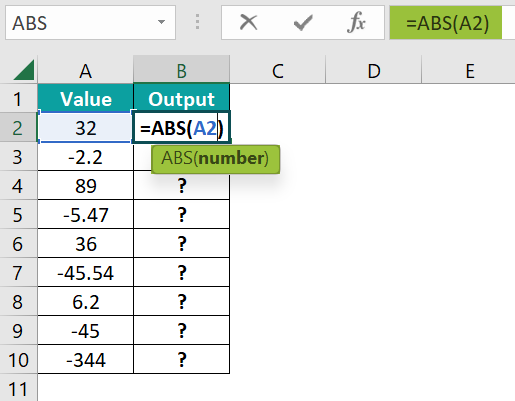2. Press the “Enter” key. The result is “32”, as shown below.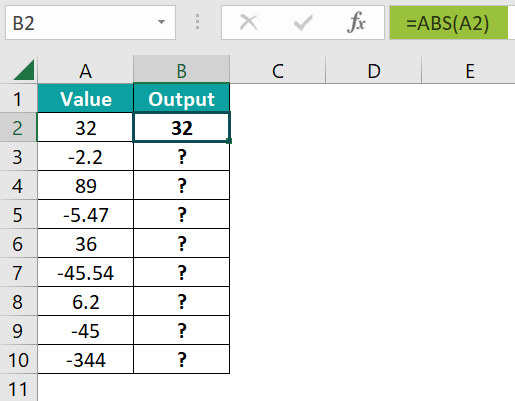3. Drag the formula from cell B2 to B10 using the fill handle. The output is shown below.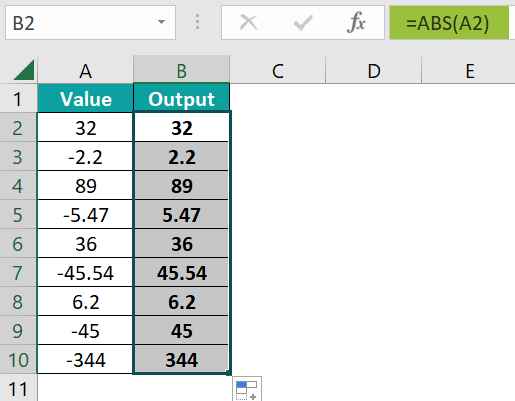### Examples

We will understand some advanced scenarios using the ABS Excel function examples.

#### Example #1

We will find the absolute value from the given values using the ABS function.

In the table, the data is,

• Column A contains the Description of the formula.
• Column B displays the Output.

The procedure to find the value using the ABS formula is,

Enter the formula =ABS(10) in cell B3, =ABS(-10) in cell B4, =ABS(A1) in cell B5, and press “Enter” after entering each formula.

We get the output shown above.

#### Example #2

We will first subtract the numeric values and then find the absolute value of the subtracted results using the ABS function.

In the table, the data is,

• Column A contains Value 1.
• Column B contains Value 2.
• Here, column C contains the result of Subtraction.
• Column D displays the ABS Result.

The steps to find the value using the ABS formula are,

• Step 1: Select cell C2, enter the formula =A2-B2, and press the “Enter” key. The result is ‘13’, as shown below.
• Step 2: Drag the formula from cell C2 to C5 using the fill handle to get the subtracted results, as shown.
• Step 3:  Select cell D2, enter the formula =ABS(C2), and press the “Enter” key. The result is ‘13’, as shown below.
• Step 4: Drag the formula from cell D2 to D5 using the fill handle to get the absolute values. The output is shown below.

#### Example #3

We will calculate the sum of the given values using the SUM excel function and then find the absolute value of the sum’s result using the ABS function.

In the table, the data is,

• Column A contains the Value.
• Here, column B contains the Actual Result.
• Column C displays the ABS Result.

The steps to find the value using the ABS formula are,

• Step 1: Select cell B2, enter the formula =SUM(A2:A6), i.e., the sum of the values in the cell range A2:A6, and press the “Enter” key. The result is -2028”, as shown below.
• Step 2: Next, select cell C2, enter the formula =ABS(B2), and press the “Enter” key. The result is ‘2028’, as shown below.

[Alternatively, we can use the formula =ABS(SUM(A2:A6)) directly in the target output cell, and we will get the same result, as shown below. Cells B3 and C3 are for our reference.]

### Important Things To Note

• The “#VALUE!” error occurs if the entered number is non-numeric.
• If a cell value is empty or blank, we get the output as Zero by default.
• If the result of a formula is an error, then the ABS value of the formula results’ cell reference will also be an error.

1. What does the ABS Excel function mean?

The ABS function helps users extract only the numeric part of a negative cell value and not its sign.

For example, we will calculate the average of the given values and then find the absolute value of the average result using the function.

In the table, the data is,
• Column A contains the Value.
• Here, column B contains the Actual Result.
• Column C contains the ABS Result.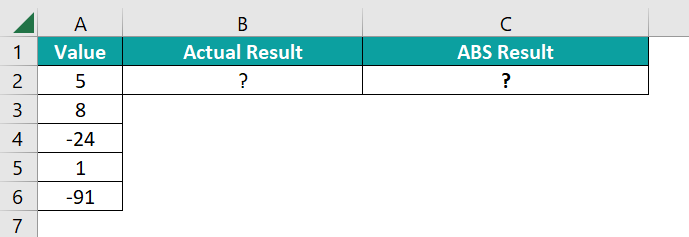The steps to find the value using the ABS formula are,

Step 1: Select cell B2, enter the formula =AVERAGE(A2:A6), and press the “Enter” key. The result is ‘-20.2’, as shown below.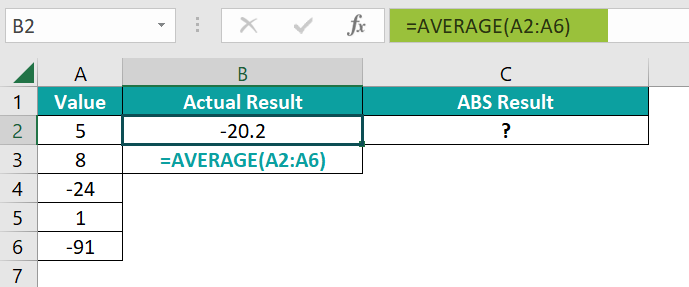Step 2: Next, select cell C2, enter the direct formula =ABS(AVERAGE(A2:A6)) or =ABS(B2), and press the “Enter” key.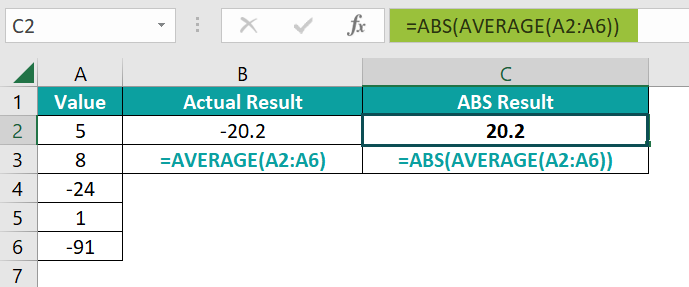The result is “20.2”, as shown above. Cells B3 and C3 are for our reference.

2. How to insert the ABS Excel function?

We can insert the ABS function as follows:
1) Select an empty cell for the output.
2) Type =ABS( in the selected cell. [Alternatively, type =A and double-click the ABS function from the list of suggestions shown by Excel.]
3) Enter the arguments as cell values or cell references and close the brackets.
4) Press the “Enter” key.

For example, we will calculate the sum-product of the given values and then the absolute value of the sum-product result using the ABS function.

In the table, the data is,
• Column A contains the Value.
• Here, column B contains the Actual Result.
• Column C contains the ABS Result.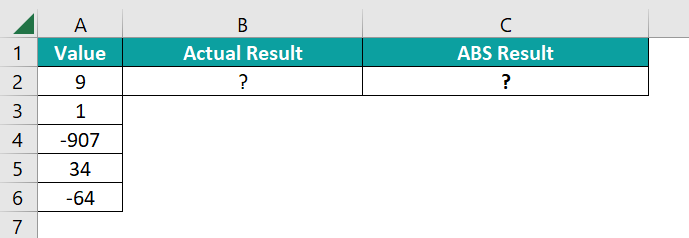The steps to find the value using the ABS formula are,

Step 1: Select cell B2, enter the formula SUMPRODUCT(A2:A6), and press the “Enter” key. The result is ‘-927’, as shown below.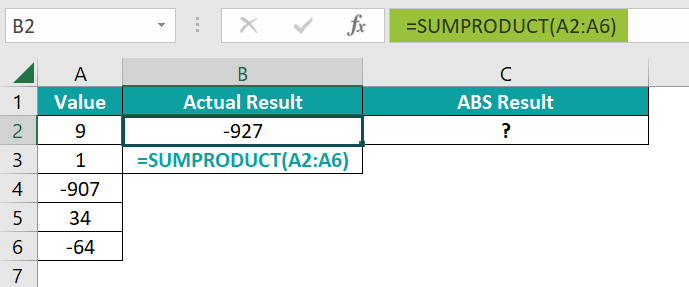Step 2: Next, select cell C2, enter the direct formula =ABS(SUMPRODUCT(A2:A6)) or =ABS(B2), and press the “Enter” key.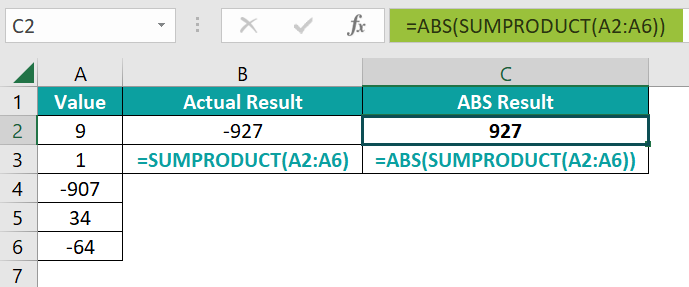The result is “927”, as shown above. Cells B3 and C3 are for our reference.

3. Where is the ABS Excel function?

The ABS function is in the “Formulas” tab, as shown below.

Choose an empty cell for the output → select the “Formulas” tab → go to the “Function Library” group → click the “Math & Trig” drop-down à→ select the “ABS” function, as shown below.